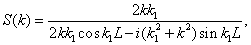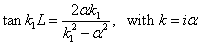# Physics 751 Homework #5

Due October 1, 11:00 am.

1. (a) For the finite square well potential V = 0 for x < 0, V = -V0 for 0 <  x  < L, V = 0 for L < x, prove that the transmission amplitude S(k) for an electron coming in from the left with momentum k iswhere k1 is the momentum inside the well. Compare this with the transmission through a square barrier in http://www.phys.virginia.edu/classes/751.mf1i.fall02/OneDimSchr.htm  and comment on the similarities and differences.

(b) Sketch the probability of transmission carefully as a function of energy, or plot it with Maple or Mathematica. For what energies is there perfect transmission?  Can you give any physical explanation?

(c) Now regard the incoming momentum k as a complex variable. Note that S(k) become infinite whenand use the formulato show that this is equivalent to the formulas for bound states in the finite square well.  Give a physical interpretation of why an infinite value of S(k) would correspond to a bound state.

Shankar problems:  5.2.1, 5.2.2.